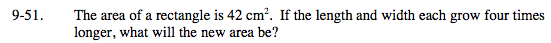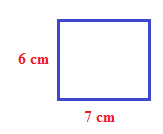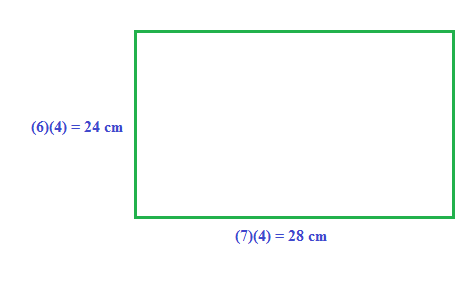Home > CC2MN > Chapter mc29 > Lesson mc29.2.2 > Problem9-51

9-51.

The area of a rectangle is 42 cm2. If the length and width each grow four times longer, what will the new area be? Homework Help ✎Make an example of a rectangle with an area of 42 cm2.
Since the area of a rectangle is (base)(height), which two numbers multiplied together gives 42?Multiply base and height by 4Now find the area of the larger rectangle by multiplying the new base and the new height.